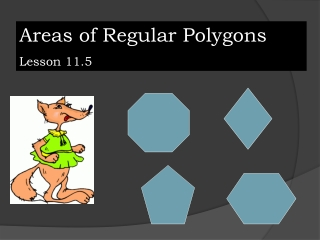DownloadDownload PresentationAreas of Regular Polygons Lesson 11.5

# Areas of Regular Polygons Lesson 11.5

Download Presentation## Areas of Regular Polygons Lesson 11.5

- - - - - - - - - - - - - - - - - - - - - - - - - - - E N D - - - - - - - - - - - - - - - - - - - - - - - - - - -
##### Presentation Transcript

1. Areas of Regular Polygons Lesson 11.5

2. Equilateral Triangle Remember: drop an altitude and you create two 30-60-90 triangles. What is the measure of the sides and altitude in terms of one side equaling s? Altitude = s√3 2

3. C Given: ∆ CAT is equilateral, and TA = s Find the area of ∆CAT A T S A∆CAT = = = 2

4. Theorem 106: Area of an equilateral triangle = the product of 1/4 the square of a side and the square root of 3. Where s is the length of a side Aeq∆= 2

5. An equilateral triangle has a side of 10 cm long. Find the area of the triangle. A = 102(√3) 4 A = 25√3 cm2

6. Area of a regular polygon: Remember all interior angles are congruent and all sides are equal. N Regular pentagon: O is the center OA the radius OM is an apothem T E O P M A

7. You can make 5 isosceles triangles in a pentagon. Any regular polygon: Radius: is a segment joining the center to any vertex Apothem: is a segment joining the center to the midpoint of any side.

8. Apothems: All apothems of a regular polygon are congruent. Only regular polygons have apothems. An apothem is a radius of a circle inscribed in the polygon. An apothem is the perpendicular bisector of a side. A radius of a regular polygon is a radius of a circle circumscribed about the polygon. A radius of a regular polygon bisects an angle of the polygon.

9. Theorem 107: Areg poly = ½ ap Area of a regular polygon equals one-half the product of the apothem and the perimeter. Where a = apothem p = perimeter

10. A regular polygon has a perimeter of 40 cm and an apothem of 5 cm. Find the polygon’s area. A = ½ap = ½(5)(40) = 100 cm2

11. Find the area of a regular hexagon whose sides are 18 cm long. Draw the picture Write the formula Plug in the numbers Solve and label units

12. Find the perimeter Find each angle Find the apothem 18cm P = 18(6) = 108 cm Angles = 720º/6 angles = 120º per angle Radius breaks it into 60º angles. 30-60-90 triangle, apothem = 9√3 cm Write the formula, and solve.

13. A = ½ ap A = ½ (9√3)108 A = 486√3 cm 2

14. Team Challenge: A square is inscribed in an equilateral triangle as shown. Find the area of the shaded region.

15. 2x + x√3 = 12 x = 12 2 + √3 x = 12(2 – √3) A (shaded) = ½ (12)(6√3) – [12(2 – √3)√3]2 = 1764√3 - 3024 x√3 x x√3 x